# X/Y Toolhead Offsets Calibration

Model [] AON-M2 [] AON-M2 2020 [] AON M2+ [] AON M2+ (CE)
Category [ ] Preventive [ ] Corrective [] Calibration

## Summary

The procedure that follows gives instructions on how to calibrate the T1 relative positions along the X/Y-axes with the T0. Correctly calibrated X/Y toolhead offsets can prevent shifts in prints caused by misaligned toolheads and/or toolhead collision(s).

Estimated time: 30-45 minutes

## Tools

Qty Description Specification
1 Parts Removal Tool Plastic

None required.

## Personal Protective Equipment

Qty Description Minimum Specification
1 Safety Eyewear ANSI/ISEA Z87.1
1 Heat-resistant Gloves EN 407-2004

## Software Requirements

Use the Graphical User Interface (GUI)/web browser to confirm the software version that is installed on your AON3D machine:

1. Select Settings > Updates.

If your machine does not have the latest software version, refer to the Software Update procedure.

## Preheat Build Chamber Components

Preheat all the build chamber components to the recommended temperatures before a print is started. Make sure to obey the time necessary for all machine components to gradually increase to the recommended temperature. Refer to Preheat Build Chamber Components.

## When to Calibrate the X/Y Toolhead Offsets

The X/Y toolhead offsets calibration procedure must be performed for prints that use the two toolheads on the same print model, which includes support material prints.

The X/Y toolhead offsets calibration procedure plays a normal part of operation, as small adjustments and recalibration can help with print quality.

It is common practice to calibrate the X/Y toolhead offsets as part of the setup process to make sure that the two toolheads are correctly aligned from each other along the X/Y-axes.

AON3D recommends that the X/Y toolhead offsets be calibrated when:

• The machine is moved and/or leveled.
• There is a change of ±20°C (±68°F) in component temperature(s).
• There is a change of build platform.
• Heater block assembly adjustments, removal, maintenance and/or repair occur.
• Hot end adjustments, removal, maintenance and/or repair occur.

## X/Y Toolhead Offsets Calibration Files

AON3D provides X/Y offset scale calibration files to help with the X/Y toolhead offsets calibration process. The .zip files must be imported/extracted to a computer and correctly oriented onto the Simplify3D® build plate. For best results, make sure to use the same processes on the scale files as in your actual print when a .gcode file is made.

Four X/Y offset scales are included in the zip file:

• 0.4 mm nozzle: 0.1 mm (0.004 in.)
• 0.4 mm nozzle: 0.2 mm (0.008 in.)
• 0.6 mm nozzle: 0.1 mm (0.004 in.)
• 0.6 mm nozzle: 0.2 mm (0.008 in.).

Use the nozzle orifice diameter guidelines as follows:

• Nozzles 0.25 mm to 0.4 mm: Use the 0.4 mm nozzle calibration files.
• Nozzles 0.6 mm to 1.2 mm: Use the 0.6 mm nozzle calibration files.

### How to use the X/Y Toolhead Offsets Calibration Files

• The X/Y calibration files are used like a vernier caliper: The set primary scale (T0), and the variable vernier scale (T1), is made as such that it is distanced at a constant fraction of the set primary scale.
• When you use the calibration scale with a vernier constant of 0.2 mm, each line on the vernier scale is distanced 0.2 mm apart from the point of origin, which is found at the center of the printed scale (T0).
• Once printed, one of the lines on the variable vernier scale (T1) should line up with the set primary scale (T0). To calculate the offset, count the line(s) from the point of origin to the mark that lines up with the fixed scale and multiply this by the vernier constant.
• Lines to the left of the point of origin are negative offsets. Lines to the right are positive offsets.
• All X/Y offset adjustments are made to T1.

The printed calibration scale has the results shown below. With the 0.2 mm vernier constant, the user would calculate the offset adjustments as follows: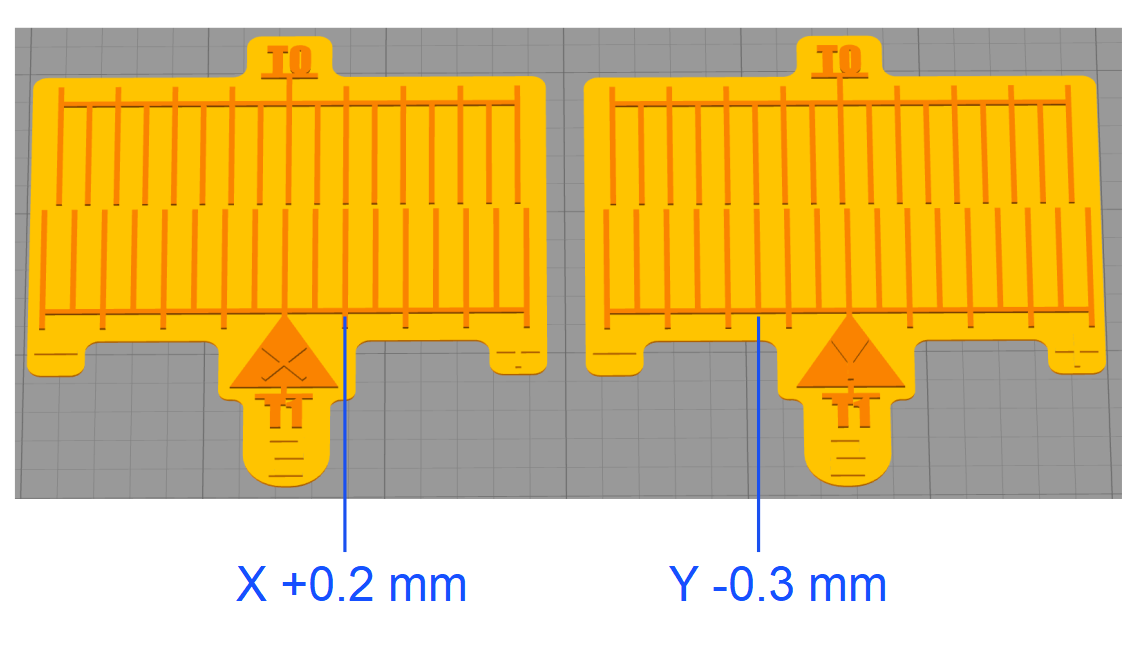#### Examples

X-Axis Scale:

• The T0 and T1 printed lines are aligned in the positive vernier constant (alignment occurs to the right from the point of origin), which results to a positive value of 0.2 mm.
• There are a number of two lines indicated on the printed scale from the point of origin, which give us a value of 2.
• The formula is as follows: (0.2 x 2) = 0.4 X-axis offset on T1.

Y-Axis Scale:

• The T0 and T1 printed lines are aligned in the negative vernier constant (alignment occurs to the left from the point of origin), which gives us a negative value of -0.2 mm.
• There are a number of three lines indicated on the printed scale from the point of origin, which give us a value of 3.
• The formula is as follows: (-0.2 x 3) = -0.6 Y-axis offset on T1.## X/Y Calibration Procedure

1. Load filament into the two toolheads. Refer to Load Filament.
2. Calibrate the two Z-axis probes. Refer to the Z-Axis Calibration Index section, and select your AON3D machine model.

### Calibration Files Preparation

1. Two sets of calibration files are provided:

• One set with a vernier constant of 0.1 mm.
• One set with a vernier constant of 0.2 mm.

3. Import the files that follow into Simplify3D®:

• X - 0.2 mm offset scale - 0.6 mm nozzle (BASE).stl
• X - 0.2 mm offset scale - 0.6 mm nozzle (T0).stl
• X - 0.2 mm offset scale - 0.6 mm nozzle (T1).stl
• Y - 0.2 mm offset scale - 0.6 mm nozzle (BASE).stl
• Y - 0.2 mm offset scale - 0.6 mm nozzle (T0).stl
• Y - 0.2 mm offset scale - 0.6 mm nozzle (T1).stl
4. In Simplify3D®, select the three X-axis models and perform the operations that follow:

• Select Edit > Group Selection.
• Select Edit > Align Selected Model Origins.
• Move the grouped X-axis models to the necessary location onto the print surface.
5. In Simplify3D®, select the three Y-axis models and perform the operations that follow:

• Select Edit > Group Selection.
• Select Edit > Align Selected Model Origins.
• Move the grouped Y-axis models to the necessary location on the print surface.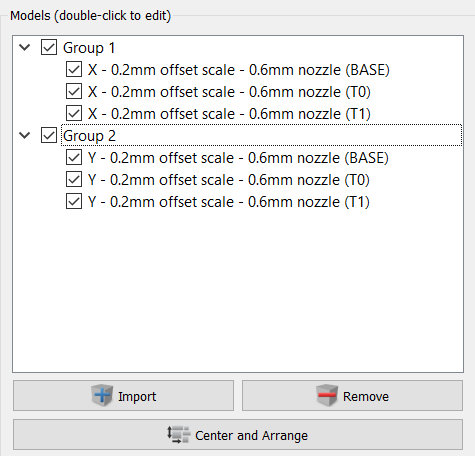6. Make sure that the X-axis and the Y-axis offset scales are oriented as shown in the figure below. Make sure that the conditions that follow are met:

• The X-axis offset scale symbol + points towards the front right side of the machine.
• The Y-axis offset scale symbol + points towards the rear of the machine.
• Set the scale factor to 100%.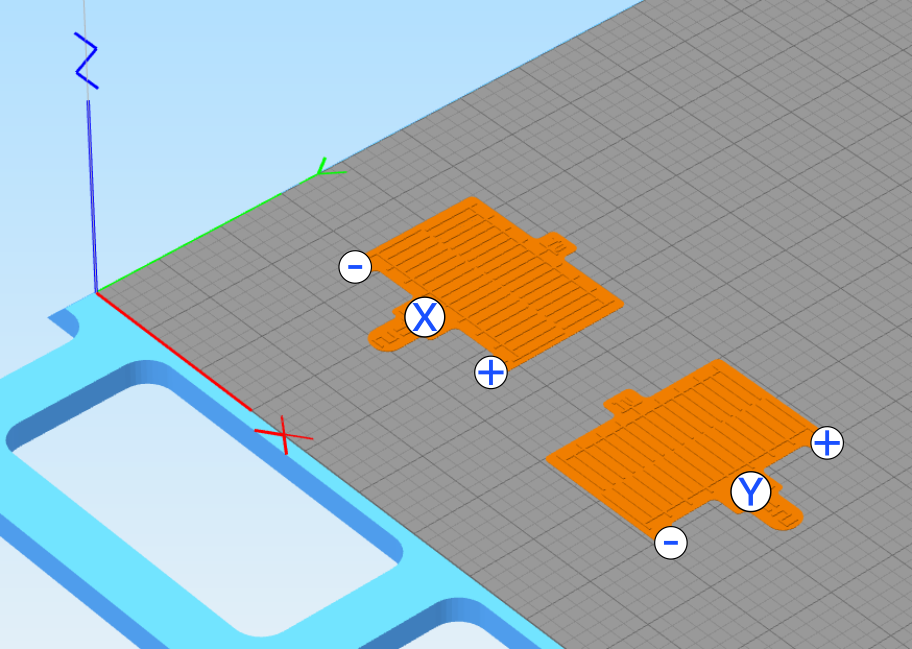7. Select the process that will be used for T0, and select the four models that will use this process.

8. Make sure that T0 is selected as the primary extruder for the layer.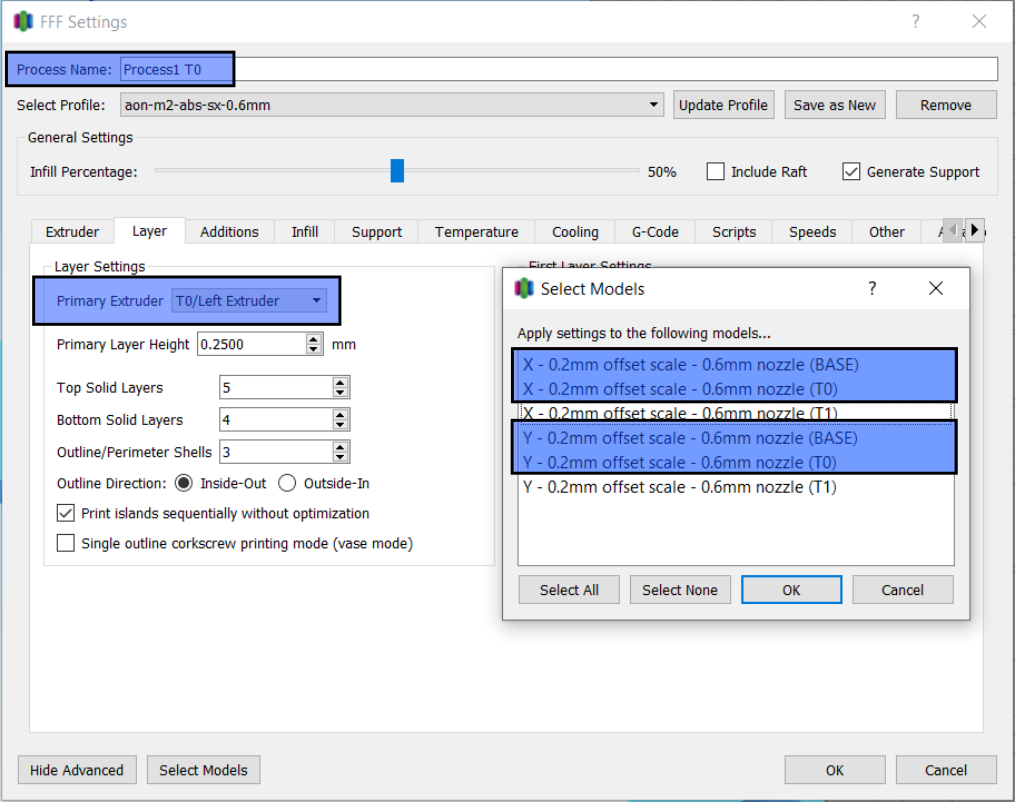9. Select the process that will be used for T1, and select the two models that will use this process.

10. Make sure that the primary extruder for the layer is T1.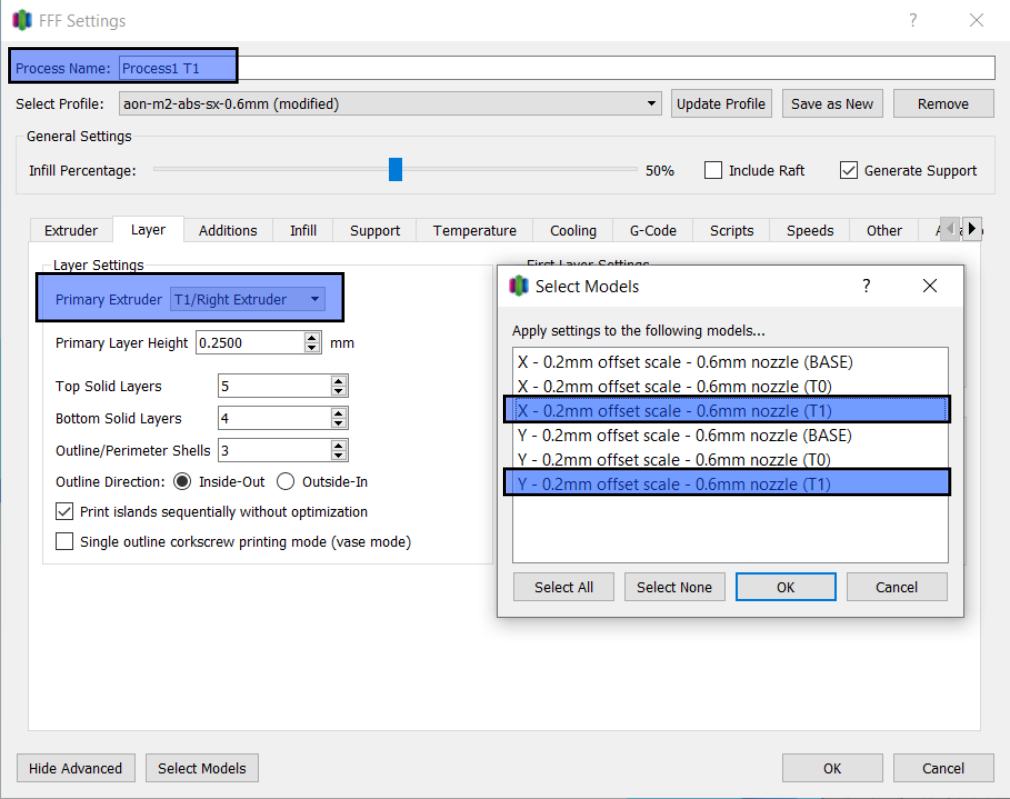11. Select Prepare to Print!, select the two processes, and select Continuous Printing: layer-by-layer.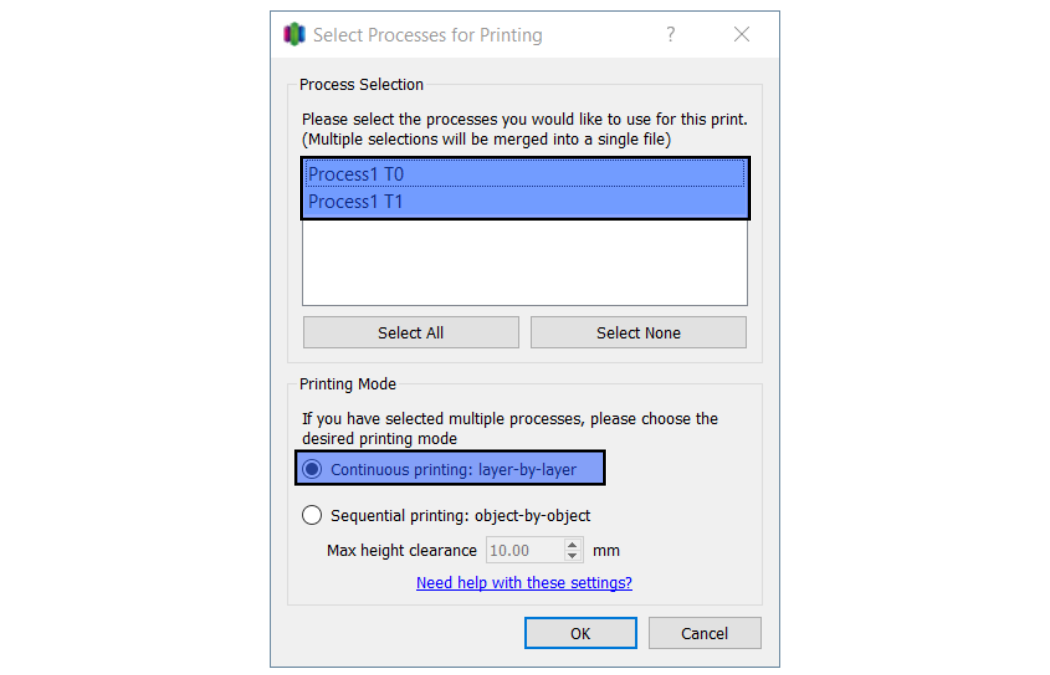12. Review the process preview. Make sure that the T1 process is only used for the T1 scale.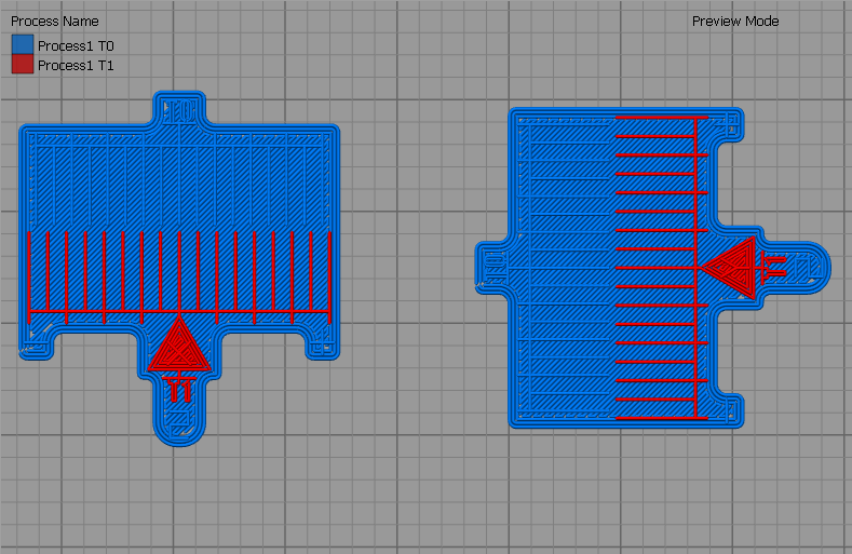13. Select Save to generated the .gcode file.

14. Upload the file to the machine.

### X/Y Toolhead Offsets Calibration Procedure

• All units of measurement are metric (mm).
• Machine default offset values are 0 mm for the X/Y axes.
• Offset calibration values must not be more than 5 mm. An offset of ±2 mm in the X/Y axes is typical.

### XY Calibration Screen Entry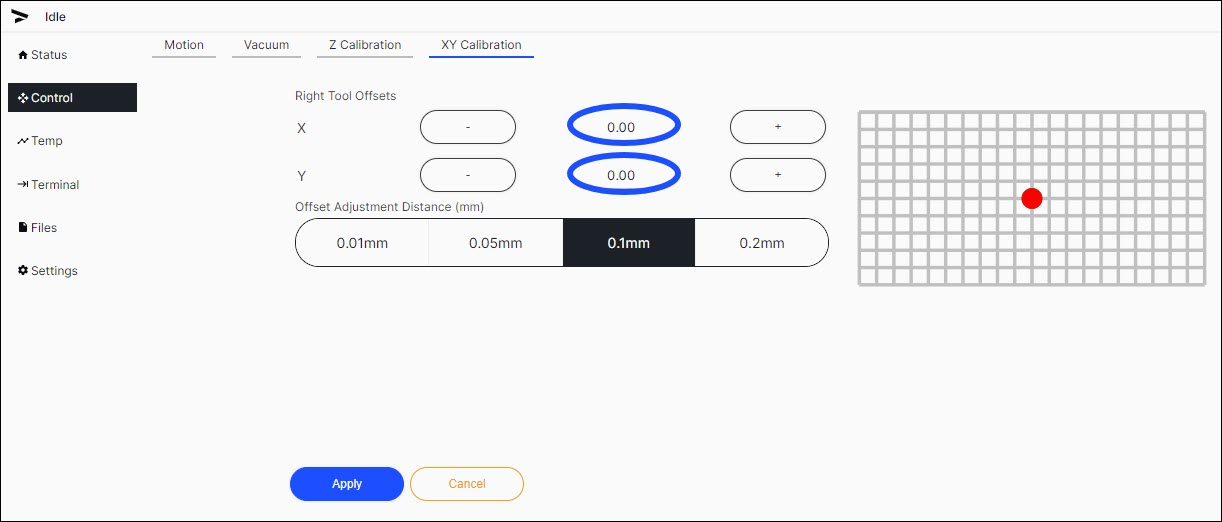1. Select Temp, and enter the target temperatures for the two toolheads.
2. Let the two toolheads get to the target temperatures.
3. Select Files > Print found next to the calibration print .gcode file.
4. When the print is complete, open the build chamber door, and use the parts removal tool to remove the printed scale from the build print surface. Make sure to close the build chamber door as to not let the build chamber temperature decrease.
5. Examine the results. If the T0 and T1 center lines are not aligned with each other, examine the lines to calculate the necessary T1 offset(s) to align the two toolheads.

#### First X/Y Calibration Attempt

1. The first printed scale gives the information that follows:
• X-axis: The T0 and T1 align at seven lines to the left (negative value) from the point of origin (T0).
• The formula is as follows: -0.2 x 7 = -1.4 offset is necessary on the T1 X-axis.
• Y-axis: The T0 and T1 align at two lines to the right (positive value) from the point of origin (T0).
• The formula is as follows: 0.2 x 2 = 0.4 offset is necessary on the T1 Y-axis.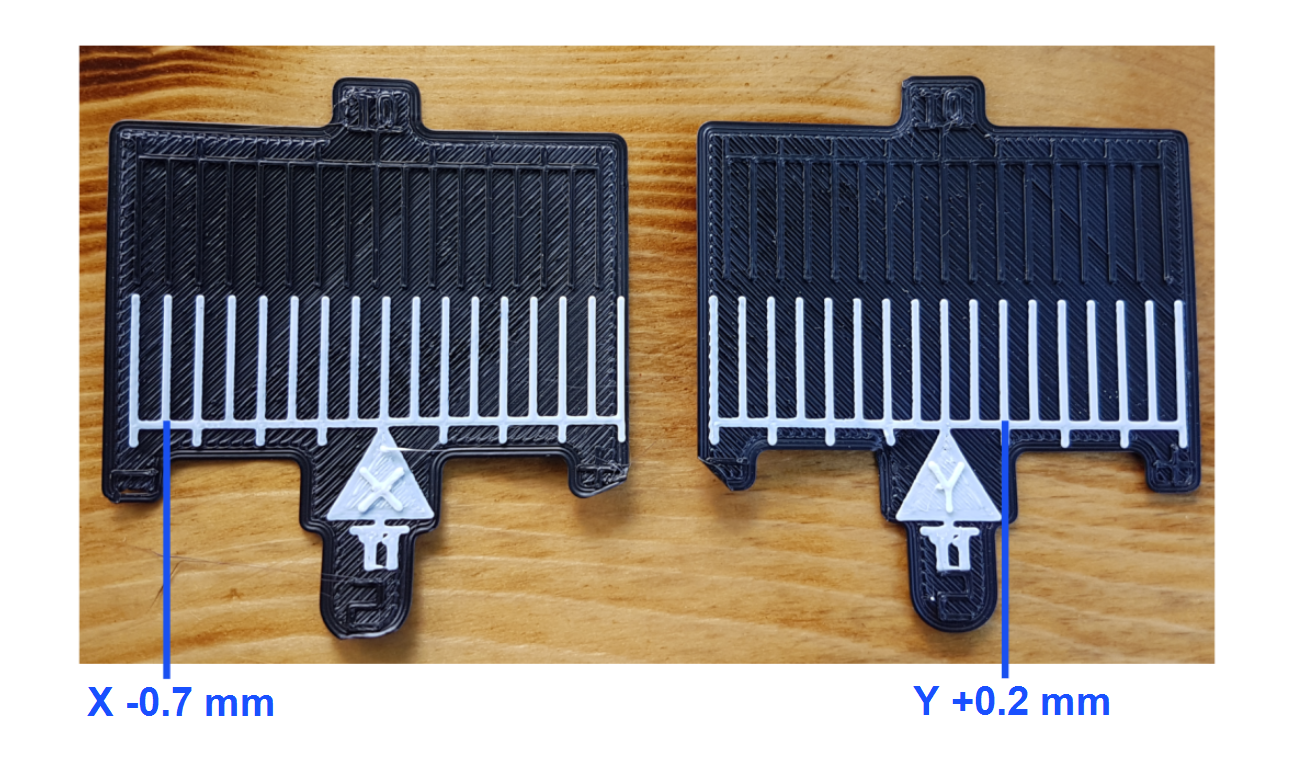2. Select Motion > XY Calibration.
3. Select `0.1mm` as the `Offset Adjustment Distance (mm)`.
4. Use the `-` button to input `1.4` on the X-axis.
5. Use the `+` button to input `0.4` on the Y-axis.
6. Select `Apply` to save the offsets.
7. Print the 0.2 vernier constant calibration print .gcode file.

#### Second X/Y Calibration Attempt

1. The second printed scale gives the information that follows:
• X-axis: The T0 and T1 lines align at one line to the left (negative value) from the point of origin (T0).
• The formula is as follows: -0.2 x 1 = -0.2 offset is necessary on the T1 X-axis.
• Y-axis: The T0 and T1 lines align at the point of origin (T0).
• The formula is as follows: (0.2 x 0) = 0 offset necessary on the T1 Y-axis. The Y-axis offset value remains the same, and is now correctly calibrated.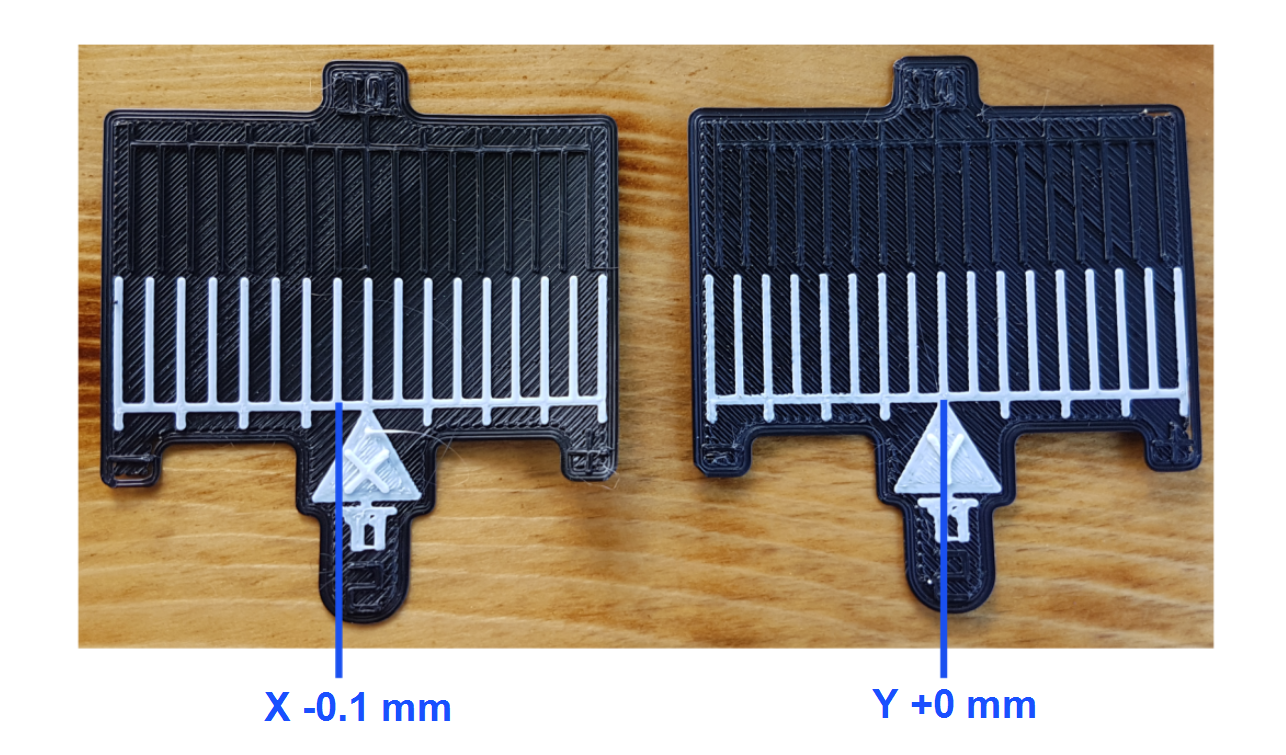2. Select Motion > XY Calibration.
3. Use the `-` button to input `0.2` on the X-axis.
4. Select `Apply` to save the offsets.
5. Print the 0.2 vernier constant calibration print .gcode file.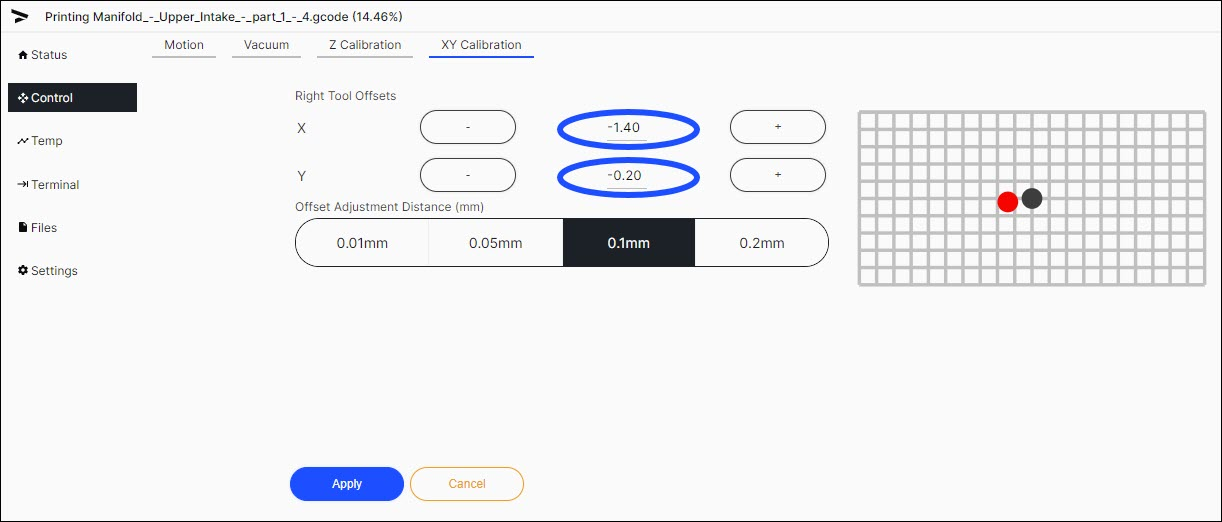#### Third X/Y Calibration Attempt

1. The third printed scale gives the information that follows:
• X-axis: The T1 tool has printed 0.0 when compared to T0.
• Y-axis: The T1 tool has printed 0.0 when compared to T0.
2. Adjustments are no longer necessary at this point.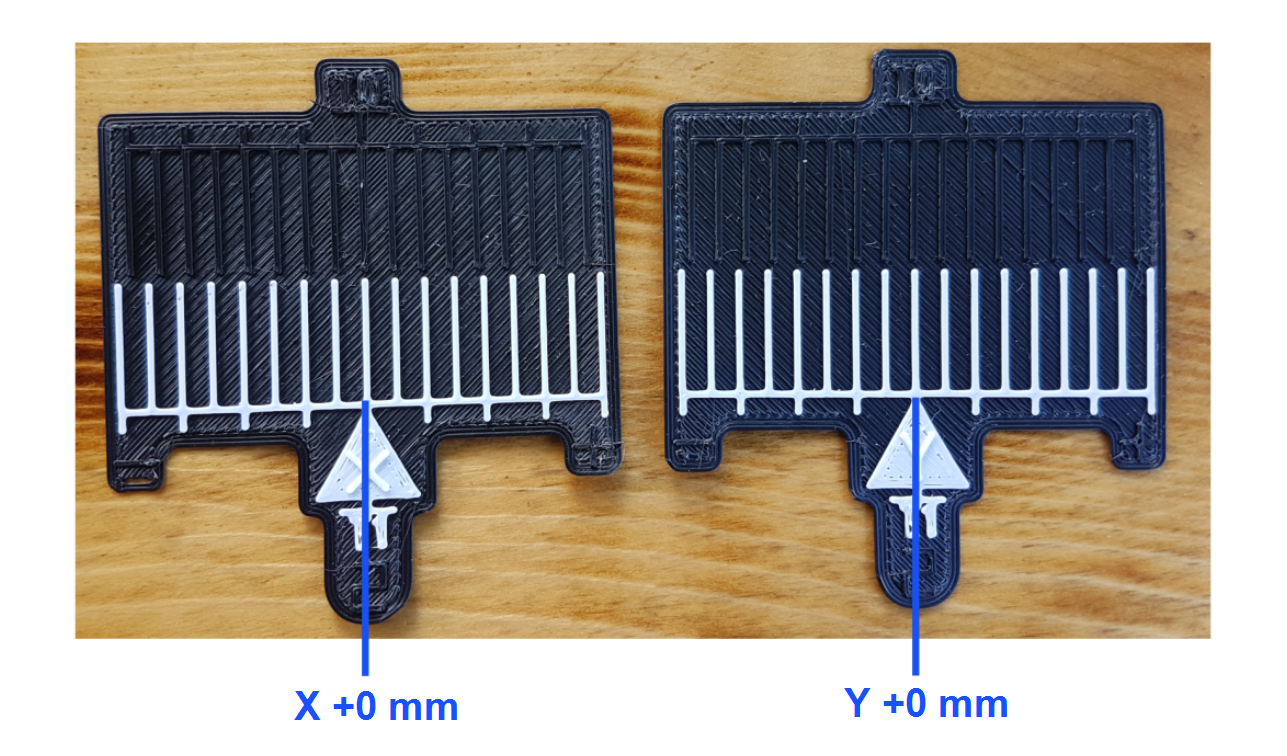### Terminal Screen Entry

The `M218` M-Code command can be used to read and apply offsets to the selected toolhead in the X/Y axes.

• The use of `M218 T1` reads the T1 X/Y offsets. No changes to offsets are made with the use of this command.
• The use of `M218 T1 X0.02 Y0.03` changes, and saves, the X/Y axes offsets.

For more information on G-codes and M-codes, please refer to Machine Codes Guide.

1. On the GUI, go to Terminal, and input `M218 T1`.

2. Note the X/Y offset values. These are the T1 X/Y offsets that are saved in the firmware.

3. Go to Temp, and input the target temperatures for the two toolheads.

4. Let the two toolheads get to the target temperatures.

5. Select Files > Print that are next to the calibration print .gcode file.

6. When the print is complete, open the build chamber door, and use the parts removal tool to take out the printed scale from the build print surface. Make sure to close the build chamber door as to not let the build chamber temperatures decrease.

7. Examine the results. If the T0 and T1 center lines are not aligned with each other, examine the lines to calculate the necessary T1 offset(s) to align the two toolheads.

8. Calculate the new T1 offset(s) as follows:

• New X offset = Current X-axis offset + Measured X-axis offset.
• New Y offset = Current Y-axis offset + Measured Y-axis offset.
9. To apply the adjusted T1 X and Y tool offsets, make sure the print has stopped, and enter `M218 T1 X[X offset] Y[Y offset]` in the Terminal tab.

10. The machine will automatically home the axis(axes) that was(were) adjusted and save the offset(s).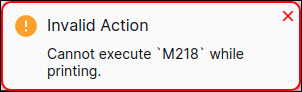11. Do the calibration procedure until results are satisfactory between the print position between the two toolheads.

12. The T1 Z-offset must be calibrated. Refer to the Z-Axis Calibration procedure.

#### First X/Y Calibration Attempt

1. The first printed scale gives the information that follows:
• X-axis: The T0 and T1 align at seven lines to the left (negative value) from the point of origin (T0).
• The formula is as follows: -0.2 x 7 = -1.4 offset is necessary on the T1 X-axis.
• Y-axis: The T0 and T1 align at two lines to the right (positive value) from the point of origin (T0).
• The formula is as follows: 0.2 x 2 = 0.4 offset is necessary on the T1 Y-axis.2. Select Terminal, and enter `M218 T1 X-1.4 Y0.4` to apply the new T1 X and Y offsets.
``````M218 T1 X-1.4 Y0.4
``````
3. The machine will automatically home the X/Y-axes and save the offsets.
4. To confirm that the new X/Y offsets were successfully saved to T1, input the `M218 T1` command.
``````< M218 T1
> Hot end offsets: X:-1.4 Y:0.4
``````
5. Print the 0.2 vernier constant calibration print .gcode file.

#### Second X/Y Calibration Attempt

1. The second printed scale gives the information that follows:
• X-axis: The T0 and T1 lines align at one line to the left (negative value) from the point of origin (T0).
• The formula is as follows: -0.2 x 1 = -0.2 offset is necessary on the T1 X-axis.
• Y-axis: The T0 and T1 lines align at the point of origin (T0).
• The formula is as follows: (0.2 x 0) = 0 offset necessary on the T1 Y-axis. The Y-axis offset value remains the same, and is now correctly calibrated.2. Calculate the new T1 tool offsets as follows:
• New X offset: -0.2 x 1 = -0.2
• New Y offset: 0.2 x 0 = 0
3. Select Terminal, and enter `M218 T1 X-0.2` to apply the new T1 X-axis offset.
``````M218 T1 X-0.2
``````
4. The machine will automatically home the X/Y-axes and save the offset(s).
5. To confirm that the new X/Y-axes offsets were successfully saved to T1, input the `M218 T1` command.
``````< M218 T1
> Hot end offsets: X:-0.2 Y:0.4
``````
6. Print the 0.2 vernier constant calibration print .gcode file.

#### Third X/Y Calibration Attempt

1. The third printed scale gives the information that follows:
• X-axis: The T1 tool has printed 0.0 when compared to T0.
• Y-axis: The T1 tool has printed 0.0 when compared to T0.2. Adjustments are no longer necessary at this point.
3. Probe the bed with T1 to save the changes made in the probe positions in the X/Y-axes.